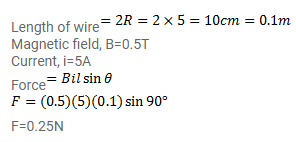# A semi-circular wire of radius 5.0cm and

Question:

A semi-circular wire of radius $5.0 \mathrm{~cm}$ and carries a current of $5.0 \mathrm{~A}$. A magnetic field B of magnitude $0.50 \mathrm{~T}$ exists along the perpendicular to the plane of the wire. Find the magnitude of the magnetic force acting on the wire.

Solution: